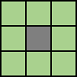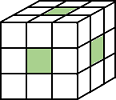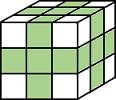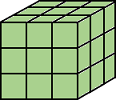# bwperim

## 语法

``BW2 = bwperim(BW)``
``BW2 = bwperim(BW,conn)``
``bwperim(___)``

## 说明

``BW2 = bwperim(BW)` 返回一个二值图像，该图像仅包含输入图像 `BW` 中对象的边界像素。如果某像素非零并且与至少一个零值像素连通，则该像素是边界的一部分。 `
``BW2 = bwperim(BW,conn)` 还指定像素连通性 `conn`。`
`不带输出参数的 `bwperim(___)` 在新图窗窗口中显示边界的二值图像。要使用此语法，`BW` 必须为二维二值图像。`

## 示例

`BW = imread('circles.png');`

`BW2 = bwperim(BW,8);`

`imshowpair(BW,BW2,'montage')``BW1 = imread('circbw.tif');`

`BW2 = bwperim(BW1);`

`montage({BW1,BW2},'BackgroundColor','blue','BorderSize',5)`## 输入参数

`4``8``6`

• 在所列方向之一上连通：内、外、左、右、上、下`18`

• 在所列方向之一上连通：内、外、左、右、上、下

• 在两个方向的组合上连通，如右下或内上`26`

• 在所列方向之一上连通：内、外、左、右、上、下

• 在两个方向的组合上连通，如右下或内上

• 在三个方向的组合上连通，如内右上或内左下